# Nuisance covariates in first-level models

Non-neuronal signals in fMRI data are caused by head motion, scanner noise, and physiological noise (e.g., cardiac and respiratory effects). For an overview, see this article. Thus, fMRI data have artifacts, and some of these can be addressed by modeling some known sources of noise when estimating the first-level (single-subject) model. This involves adding several types of nuisance covariates to the first-level design matrix. Previously, we talked about adding high-pass filter covariates. Other types include:
• Estimates of head movement (and transformations based on these)
• Dummy or "spike" regressors to model images that are likely outliers
• Signals from regions assumed to contain no true signal but capture spatially distributed noise, like the ventricles (CSF space)
Here, we'll go over some of the basics of including nuisance covariates. for this, we'll use fMRI data from a sample subject, so we're working with real motion estimates. You’ll need a folder called Pinel_data_sample_subject on your Matlab path.

### Orientation to Pinel data files

The Pinel Localizer task was designed to probe several different types of basic cognitive processes, such as visual perception, finger tapping, language, and math. Several of the tasks are cued by reading text on the screen (i.e., visual modality) and also by hearing auditory instructions (i.e., auditory modality). The trials are randomized across conditions and have been optimized to maximize efficiency for a rapid event related design. There are 100 trials in total over a 5-minute scanning session. Read the original paper for more specific details about the task and the dataset paper.
This dataset is well suited for these tutorials as it is (a) publicly available to anyone in the world, (b) relatively small (only about 5min), and (c) provides many options to create different types of contrasts. There are a total of 94 subjects available.
Our Pinel_data_sample_subject is from an individual collected at Dartmouth's Brain Imaging Center (DBIC). It is organized in a particular standardized file format called BIDS (Brain imaging Data structure). The whole dataset is available in BIDS format from Luke Chang's g-node GIN repository.
To have a look at this, let's go to the Pinel_data_sample_subject folder. Drag and drop it into your Command Window, or drag the folder into Terminal (Mac) and copy the path, then use the cd( ) command in Matlab. e.g.,
% This works for me, but you can't copy it exactly unless you have my laptop! Which i hope is not the case.
cd('/Users/torwager/Dropbox (Dartmouth College)/COURSES/Courses_Dartmouth/2021_3_Spring_fMRI_Class/Labs_and_Lectures_for_Instructors/Labs_and_assignments/Lab_5/Pinel_data_sample_subject')
Now, let's look at the files:
ls % list files
The main files we're interested in are:
The image data in 4-D Nifti format
The task events + their onset times
The rp_.txt file is only created after realignment of the functional images, a preprocessing step. We'll assume this is done already for now (which it was for this sample participant).
After realignment with SPM software (or similar), some new files are created:
The "realignment parameters" (rp), head motion estimates, with translation and rotation for each image required to match it to the reference image (often the first image in the series or an average image)
Matlab transformation matrices describing the voxel sizes, origin (zero-coordinate), and rotation for each image
The realigned image data, with the transformations (i.e., rotation/translation) applied
To construct the design matrix for first-level analysis, we're mainly interested in two files: The task (.tsv) file that tells us how to construct the regressors of interest, and the rp*.txt file that we'll use as a set of nuisance covariates.
For further reading and information on file specifications, see the docs on fmriprep preprocessing, or this or this.

#### Create a simple design matrix from the Pinel task file

Load the realignment parameters and create a set of nuisance covariates
% Load the onsets file into a table object in matlab
% Use a CANlab function to extract the conditions (event types) and which
% events belong to each type
indic = logical(indic);
% Get a cell array of onsets for each condition
[ons, ons_with_durations] = deal(cell(1, length(names)));
for i = 1:length(names)
end
Now, we've extracted the information in a format we can use to construct a design matrix. Let's try it.
Note that we need to know the TR for this! It's 2 sec. In BIDS formatted data, this can be found in the ".json sidecar" file, in the "RepetitionTime" field. We also need the scan length in sec, which in this case is 300 sec or 5 min.
TR = 2;
X = onsets2fmridesign(ons, TR, 300);
% let's plot it
plotDesign(ons, [], TR);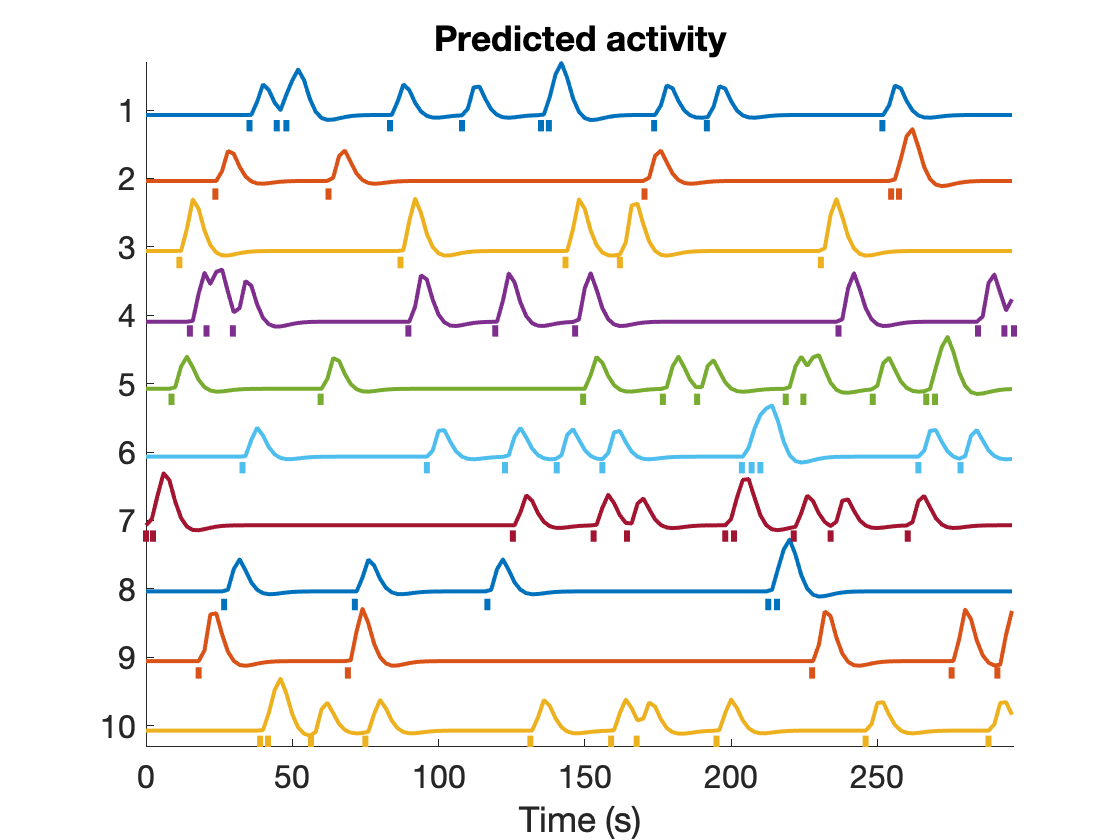This returns the design matrix, X.
We can look at the Variance Inflation Factors to see whether there are mulitcolinearity issues or not. We'll use the 'plot' option and save the handle to this figure so we can re-activate it and add things later:
fig_han = create_figure('vifs'); % Get handle to current figure
vifs = getvif(X, 0, 'plot');
Variance inflation: 1 (black line) = minimum possible (best) Successive lines indicate doublings of variance inflation factor. Red boxes have extremely high VIFs, perfect multicolinearity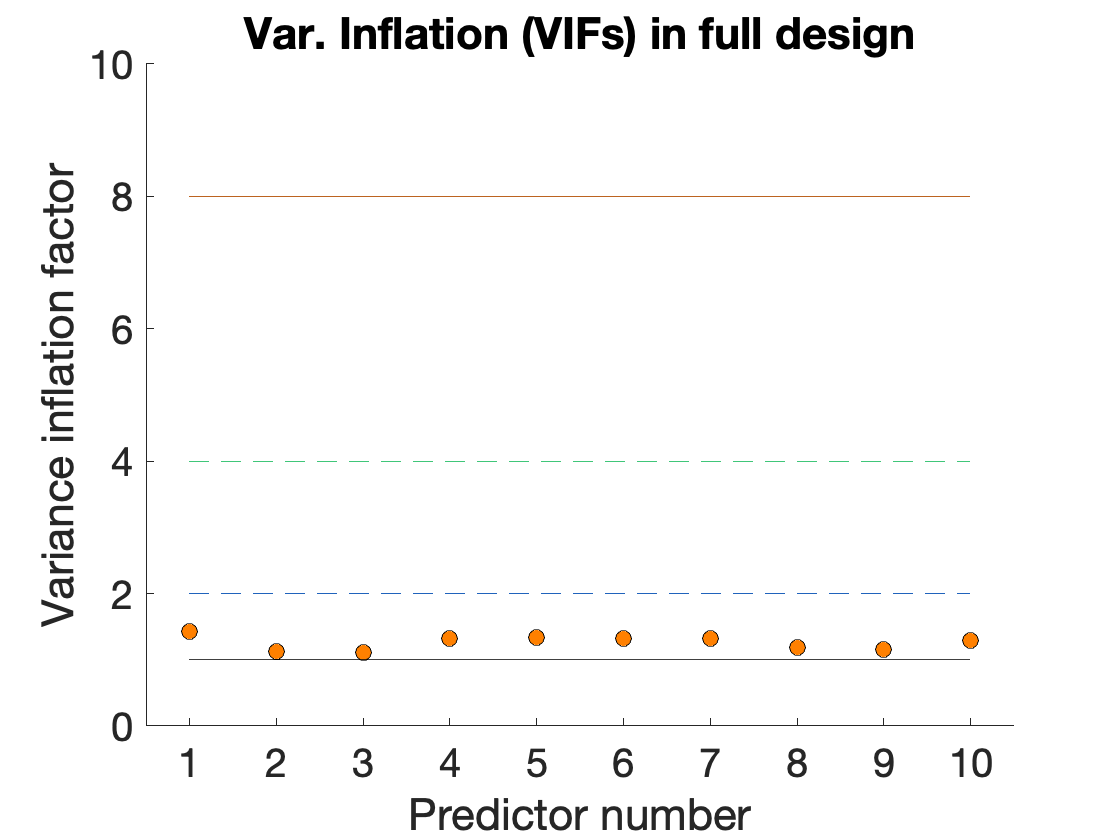1. How many conditions of interest are there?
2. Are the VIFs acceptable overall?
3. Which regressor has the highest VIF, and what condition does this correspond to?

rp = importdata(rp_file);
Let's plot them, a couple of ways. First, the standard Matlab plot:
figure; plot(rp);
legend({'x' 'y' 'z' 'roll' 'pitch' 'yaw'});
title('Movement regressors')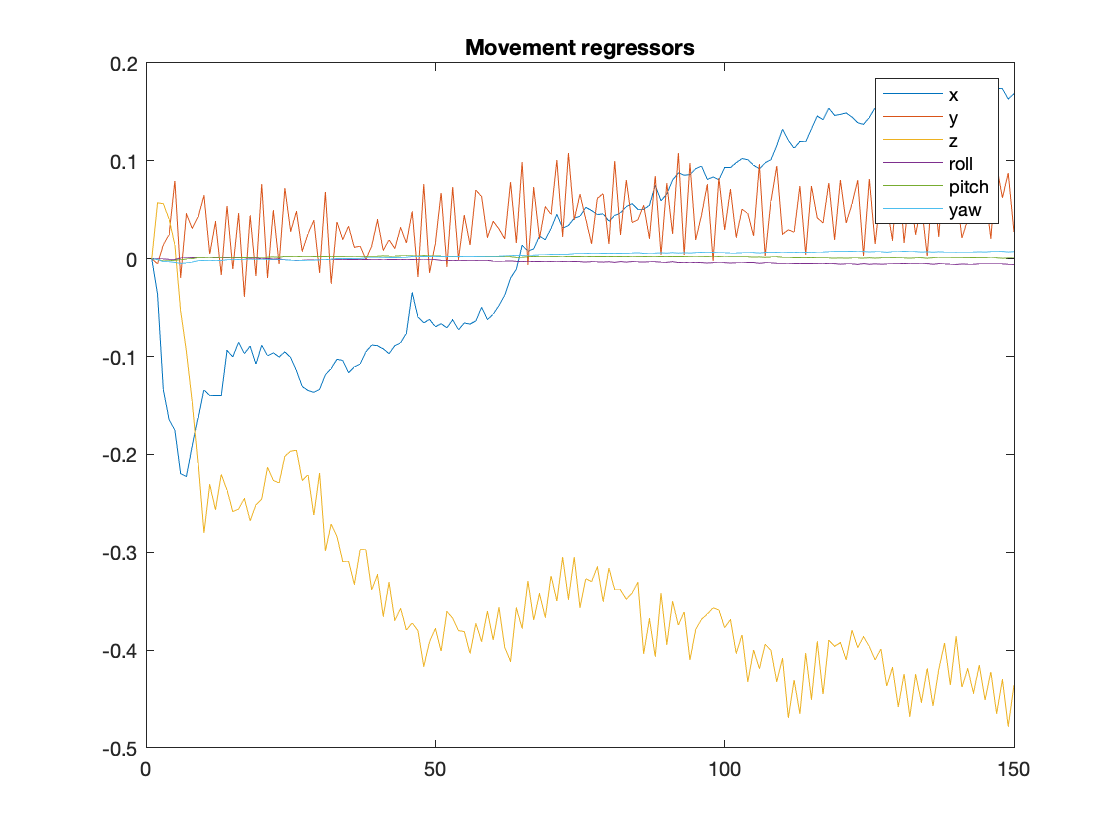We can use these as nuisance regressors. If all has gone well, we can stack these together.
X2 = [X rp];
A note on scale. The scale doesn't matter for the statistics (i.e., you can convert a regressor that measures something in units of cents into dollars by dividing by 100...the relationship with other variables won't change, and the statistics won't change. The scale of the betas (slopes) will change, but the t-values and P values will be identical.

1. Make a heat map of the full design matrix, and show it. (hint: imagesc). Can you see which are task and which are nuisance from the plot?
2. One issue with the display is that the task and nuisance parameters are on different scales. To make everything more visible, try using zscore( ) to make all the nuisance covariates (rp) mean 0 and standard deviation 1. Then re-add them to X and re-make the heat map.
3. Which movement parameter is changing most? What does this mean in terms of movement in physical space? Why might this be?
4. Which movement parameter is showing periodic motion? Why might this be?

### Parameter expansion of basic confounds

Quadratic and derivative motion regressors. The standard six-motion parameters may not account for all the variance related to head-motion. Movement in the scanner perturbs the magnetic field, creating complex nonlinear effects on the BOLD signal. Many studies include an [Friston1996] and [Satterthwaite2013] proposed an expansion of the six basic head-motion parameters, as described in, e.g., Friston et al. 1996, Lund et al. 2006, and Satterthwaite et al. 2013. The most common is to include temporal derivatives of the six base motion parameters and the quadratic (squared) terms of both rigid-body movement parameters and their derivatives, resulting in 24 head motion parameters per run. Some popular processing pipelines, like fMRIPrep, calculate this motion parameter expansion and provide it as an option for including in design matrices or regressing out of data prior to connectivity analysis.
Global signals and CompCor. Another popular set of nuisance regressors includes three global signals (csf, white_matter and possibly global gray matter). Satterthwaite et al. 2013 proposed calculating the derivatives of these and their quadratic terms as well, for a total of 36 parameters per run. Alternatively, researchers will often include principal components derived from csf and white matter, as in Behzadi et al. 2007. These form an expanded set of regressors (often 5 or more for CSF alone, for example) that are optimized to explain as much variance as possible across all voxels in the set (e.g., in CSF). Anatomical CompCor (or aCompCor) uses an anatomical mask to define csf and white matter compartments from which to extract principal components.
Issues and complications. As with all nuisance regressors, we should worry about whether this expanded set of nuisance regressors is also capturing signals of interest. This is possible because people may move in time with the task (systematically or by chance, in brief designs). In particular, global gray-matter signals track the average gray-matter response, so will inevitably be a mixture of real activation (if there is any) and noise. Others, like the physicist John Gore, have found real BOLD signals in white matter. Thus, the safest approach when including global signal components is to stick to CSF -- though even CSF could possibly contain spatially aliased signal, as pointed out by Lindquist & Wager 2008. If nuisance regressors and task regressors are correlated, the variance (uncertainty, error) in the task parameter estimates (slopes) increases, power drops, and the regression can even become unstable and yield spurious findings.
Let's expand the set of motion parameters and add those. When we calculate the quadratic terms, scale (and mean) DOES matter, so we'll zscore the regressors to center them on 0, which makes the quadratic terms capture a "U shaped" effect, orthogonal to the original rp regressors.
[~, d_rp] = gradient(rp); % gradient estimates the derivative. ~ marks an irrelevant output for us that is "thrown away"
nuis = zscore([rp d_rp]); % z-score to make each column zero-mean
nuis = [nuis zscore(quadterms)]; % z-score here for convenience in display
X3 = [X nuis];
Finally, let's re-calculate the VIFs for task regressors and add them to our original plot:
figure(fig_han) % re-activate the VIF figure
vifs3 = getvif(X3);
han = plot(vifs3(1:10), 'bo', 'MarkerSize', 8, 'MarkerFaceColor', [.5 .5 1]);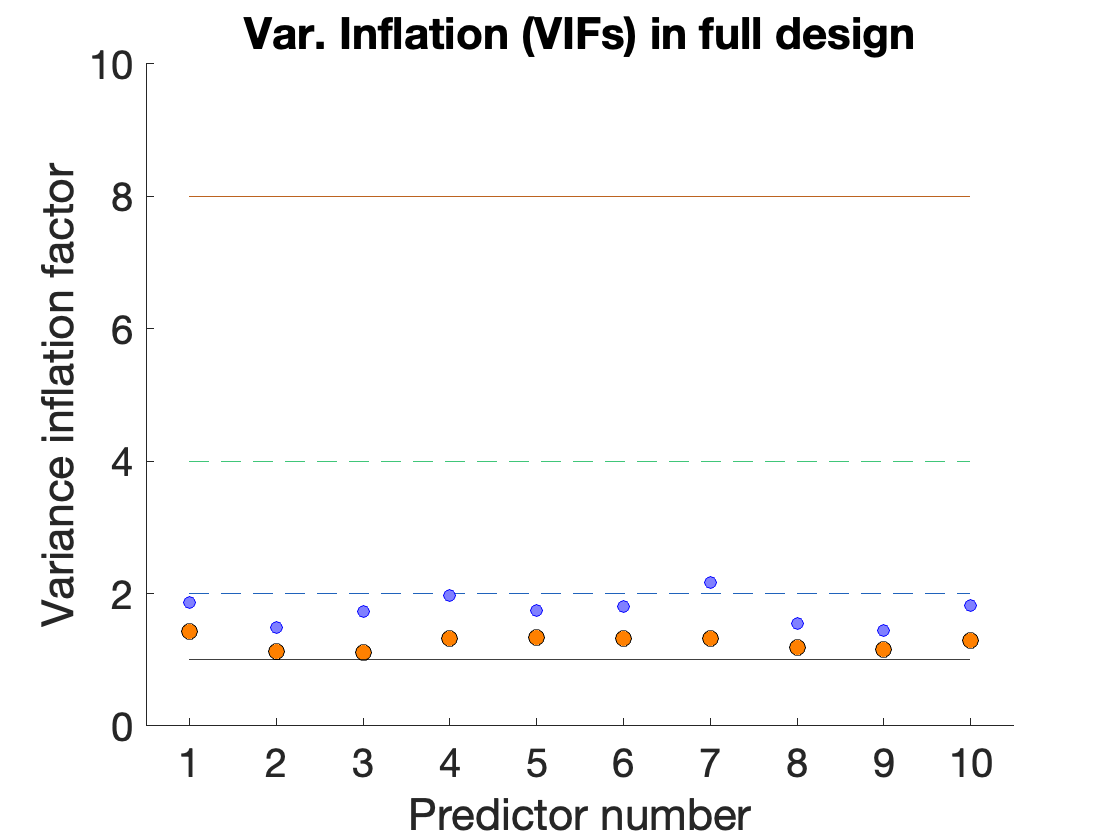1. Make a heat map of the full design matrix, and show it.
2. What's happened to the VIFs? Why?
Are the VIFs too high? There's no way to tell for sure, but we do know that we're doubling the variance (imprecision) of some of our parameter estimates. But we're reducing noise that is explained by the nuisance regressors, too.

### Outliers

Some volumes can have unusually high intensity due to lack of T1 equilibration (usually the first images in a run, defined as a series of images collected during one time the scanner is running). Volumes can also contain bad data if gradients mis-fire or are mis-timed, or if there is too much motion during the image acquisition. Such volumes can be identified and excluded as outliers if their values are unusual compared with the rest of the images in the time series. Then can then be modeled with indicator regressors (or dummy regressors, a series of 1's and 0's) for that volume. This effectively prevents the volume from contributing to the task regression parameter estimates (betas).
Here's a sample from the docs on fmriprep preprocessing for some common kinds of outliers. in the BIDS conventional structure, these would be saved in a file called desc-confounds_timeseries.tsv. We won't worry about that for now, however.
Outlier detection. These confounds can be used to detect potential outlier time points - frames with sudden and large motion or intensity spikes.
• framewise_displacement - is a quantification of the estimated bulk-head motion calculated using formula proposed by [Power2012];
• rmsd - is a quantification of the estimated relative (frame-to-frame) bulk head motion calculated using the RMS approach of [Jenkinson2002];
• dvars - the derivative of RMS variance over voxels (or DVARS) [Power2012];
• std_dvars - standardized DVARS;
• non_steady_state_outlier_XX - columns indicate non-steady state volumes with a single 1 value and 0 elsewhere (i.e., there is one non_steady_state_outlier_XX column per outlier/volume).
These methods all rely on the imaging data itself. So for this, we have to load the brain data. We'll use the CANlab fmri_data object, which is convenient to work with. Note, however, that this dataset has been realigned thus the r pre-pended to the name), but not normalized/warped to MNI space. So some functionality, like methods that extract global signal components, will not work correctly. An outlier detection method will, however, and we'll use that.
The outlier detection method is Mahalanobis distance, which is a measure of how different each image is from the rest of the images. It's based on the squared distance across images, just like the typical least-squares solution we use to fit regression models (the basis for all General Linear Models). But the Mahalanobis distance provides distances along the principal axes of variation based on the covariance across images.
Here is an intuition for why this is important. A difference of a given magnitude doesn't mean the same thing for all voxels, or for all variables in a dataset in general. If I'm classifying people in terms of their height in inches weight in lbs, and the number of fingers they have, +1 inch in height isn't so surprising, but +1 finger is. And if I transform height to feet, +1 foot is also very surprising. Rather than differences in raw units, it often makes sense to measure deviations in units of standard devations, considering the intrinsic variablilty in each variable. Furthermore, variables can move together (i.e., covary). If someone is +1 sd in height, they're also likely to be heavier than average as well, so I wouldn't want to sum the deviation in height and deivation in weight without considering their covariance and discounting it. If height and weight covary positively, +1 height and -1 weight would be much more surprising, because this pattern goes against the natural covariance in these measures. Mahalanobis distance takes care of all of that, by calculating deviations along the principal axes of covariation. Note: distances can be based on covariance or correlation. Each is sensitive to distinct effects: Covariance (the default)
The fmri_data object method mahal( ) calculates this distance for a set of images:
[D2, D2_expected, pval, wh_outlier_uncorr, wh_outlier_corr] = mahal(dat);
Retained 3 components for mahalanobis distance Expected 50% of points within 50% normal ellipsoid, found 35.33% Expected 7.50 outside 95% ellipsoid, found 7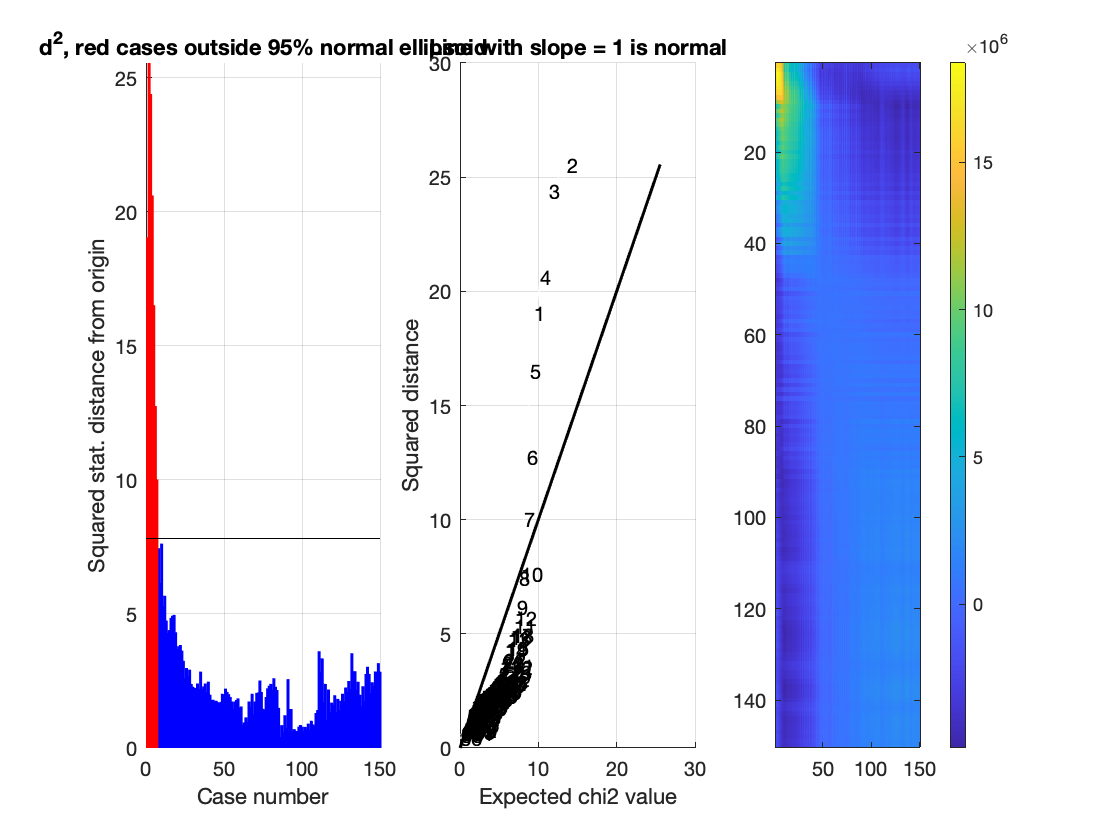Potential outliers based on mahalanobis distance: Bonferroni corrected: 4 images Cases 1 2 3 4 Uncorrected: 7 images Cases 1 2 3 4 5 6 7
The D2 output contains the Mahal values, but we're interested in which have been flagged as outliers. We'll look at wh_outlier_uncorr, which identifies images that are outside the 95% confidence region of the cloud of images (think of each image as a point) in multidimensional space. This is an indicator vector (values of 1 and 0) for which images are outliers. For a more conservative exclusion threshold, we might use wh_outlier_corr, which corrects for multiple comparisons when assessing significant deviations from the group. The plot shows us the distances for each case (image) and the relationship between the observed and expected distance. A positive deviation from the line means the image is more unusual than expected.
Let's examine the images, including some that are outliers and some that aren't. For this, we can use the get_wh_image( ) method to select some images, and then we can view slices from each.
some_images = get_wh_image(dat, [1 2 3 50 75 100]); % get 6 images, including the first 3, which are outliers
% let's use SPM to display them in orthviews
% Note: you may have to unzip this image first to get it to run! e.g., !gunzip <file name> in Matlab
spm_check_registration(V([1 2 3 50 75 100]))
% Make sure the images are displayed on the same scale:
desc = descriptives(dat);
lim = desc.prctile_vals([3 end-2]); % brightness limits: 1st and 99th percentile
spm_orthviews('Window', 1:6, lim);

1. What do you notice about which images are flagged as outliers?
2. How do they look to you? Are there noticeable differences from the other images?
3. What would it mean for a mahalanobis distance value to be usually low?

#### Completing the design matrix

These mahal values don't really look that bad visually. But we'll include them in our set anyway, by creating a unique regressor for each "bad" image. That ensures that the image value will be modeled by its own parameter, so that each outlier image can deviate from the group in a different way, and these deviations will still be captured by the "spike" regressors in the model.
It's a good idea to have a set policy for excluding images, so that there's no "fishing" post hoc (changing outlier detecfion thresholds until you get results you like... this is also called "P-hacking"). It's also a good idea to exclude only a small number of images if possible (be conservative), because if images are flagged as outliers in a way that is not random with respect to your task, this will create a selection bias that will cause systematic bias (over- or under-estimation) of your activation parameters.
This CANlab function will construct an intercept (column of ones) for one or more sessions (runs) and include a set of indicator regressors for designated outliers. We'll use find( ) in matlab to turn the logical vector of 1s and 0s in wh_outlier_corr into indices (e.g., 1, 2, 3) and construct Xn, the matrix of intercepts and dummy regressors. We'll exclude the first column of Xn because it's an intercept, and we already have one, and add the rest to the design matrix:
Xn = intercept_model(size(X, 1), find(wh_outlier_corr));
X4 = [X3 Xn(:, 2:end)];
Now let's have another look at the VIFs in our final design matrix, now in red.
figure(fig_han)
vifs4 = getvif(X4);
han = plot(vifs4(1:10), 'ko', 'MarkerSize', 8, 'MarkerFaceColor', [1 .3 .3]);
Finally, we can specify a high-pass filter matrix (if this is unfamiliar, see the previous lab on high-pass filtering) and incldue it as well:
K_input = struct('RT', TR, 'HParam', 100, 'row', ones(1, size(X, 1)));
K = spm_filter(K_input);
K = K.X0;
X5 = [X4 K];
And a final look at VIFs, in green:
vifs5 = getvif(X5);
han = plot(vifs5(1:10), 'ko', 'MarkerSize', 8, 'MarkerFaceColor', [.3 1 .3]);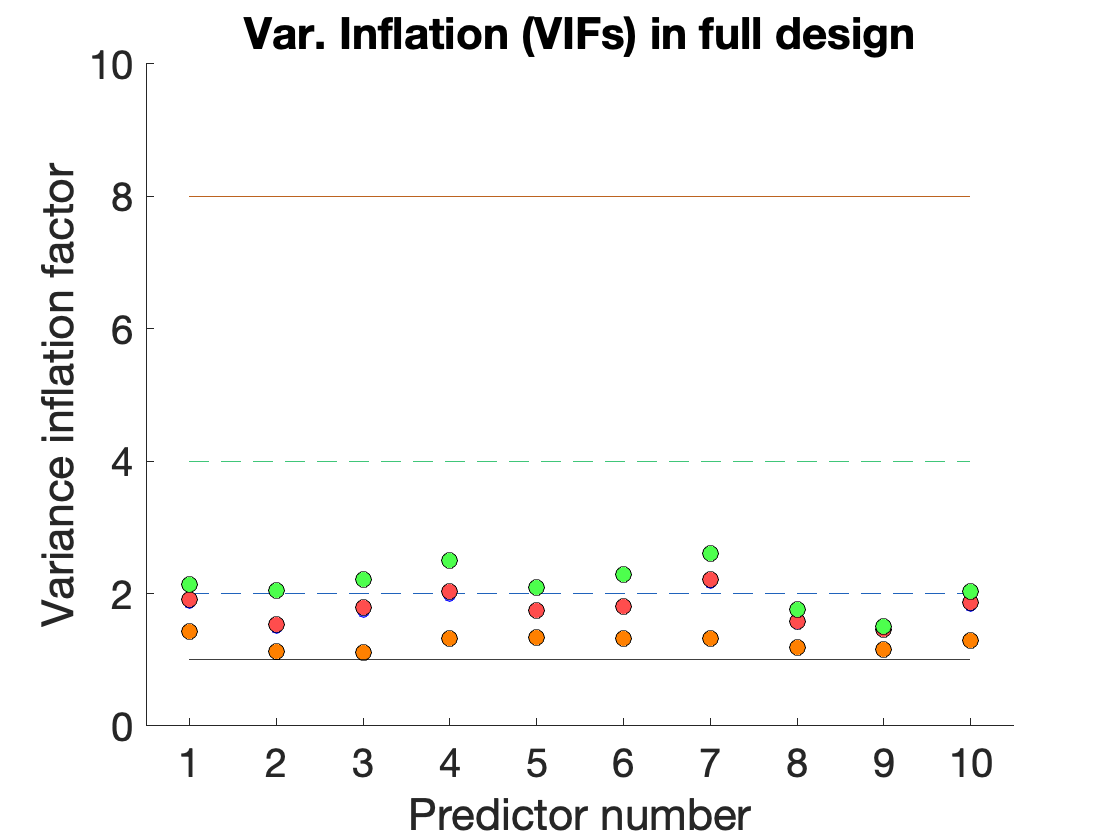Voila! Now we have a fairly complete design matrix.
This is for a one-run design (or one run of a multi-run design). For a multi-run design, we'd include separate nuisance covariates and a separate intercept for each run. Software packages generally include the intercepts automatically.
1. Using the previous lab and results on the power spectrum of the regressors of interest, what kind of high-pass filter would be appropriate? How did you come to this conclusion?

### Nuisance covariates: Wrap-up and key ideas

Here are a few key points:
• The correlations among nuisance regressors don't matter - we only care about the variance they explain jointly.
• We DO care about their covariance with the task regressors, however - and we can use VIFs to check this.
• When modeling outliers, include a separate dummy/indicator regressor for EACH outlier volume modeled.
• Excluding a large percentage of images can create selection biases that can create artifactual findings or cause true findings to be missed. Be conservative in flagging images as outliers.